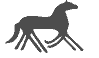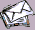Topics

Contents

GRAVITATION

Some Historical Notes will give an introduction how the knowledge on Gravitation in Space and Time has been developed over the centuries.

Classical Gravitation

In classical mechanics gravitation is described by Newton's Law of gravitation. Thus, gravitation is a field force which diminish with the square of the distance between two objects.

Whereas, Einstein's Theory of Special Relativity worked quite well with the theory of electrodynamics Newton's gravitation didn't. The problem how gravitation propagates in space was quite unclear. A new mathematical description was needed to handle the problems.

General Relativity

In his Theory of General Relativity Einstein proposed that gravitation is not a force but an intrinsic property of space and time. The effect of gravitation can be described as the curvature of the Space-Time caused by the existence of mass and energy. This introduced a new understanding the physical reality. Therefore, a new mathematical description has to be introduced as well.
This new approach now describes the gravitation by geometrical properties of the Space-Time. The 4th dimensional Space-Time is viewed as a Rieman manifold. The mathematical tool is the Tensor Algebra which describes mathematical properties in a way which is independent of the used coordinate system. This was developed by Einstein and the mathematician Hilbert. Mathematically, the effect of gravitation is described by the Einstein Field equations.The geometrical properties of Space-Time can be fully specified by the Metric Tensor G which is a 4x4 Matrix.
The properties of G are dependent on the Energy-Mass-Tensor T describing the distribution of energy and matter in space.
If there is mass, the Metric Tensor changes for each point dependent on the mass distribution. Since, mass and energy are the same they both effect the Space-Time.
If there is no mass, the Space-Time is flat and the metric tensor G looks all the same for every point. The Euclidean space is than described by the Minkowski Metric.

There is no complete solution of the Einstein field equations. Nevertheless, assuming a single object with some symmetry of the gravitational field an analytical solution is possible. The first developed solution was the Schwarzschild Solution assuming spherical symmetry. A generalization is the Kerr Solution assuming axisymmetry. All the solutions have a singularity for objects with zero radius called Black Holes.
More general solutions can only be computed via numerical simulations. If we consider yet less idealized systems the problem explodes into equations with literally thousands of terms in each equation. Some nice simulations on more general solutions can be viewed at Cyberia's General Relativity web page. In our simulations we restrict ourself to the solutions assuming symmetry.

Some Properties of General Relativity make the physical effects of gravitation which are captured by the theory much more interesting than the classical mechanics.

The Schwarzschild Solution

Schwarzschild was the first to introduce a solution of the Einstein Field Equations in 1916. His solution is only valid for a single object and the mass is distributed in such a way that the assumption of spherical symmetry holds. This will hold for point masses, spheres having a radial symmetric density and even for moving mass if the movement is radial equal distributed w.r.t. the center (e.g. an exploding, collapsing, or pulsating sphere). Thus, the equations are given in spherical coordinates. The solution is described by the Schwarzschild Metric.
An extension to the Schwarzschild Metric is the Reissner-Nordstroem Metric describing objects having mass and an electrical load.

The Birkhoffs Theorem showed that the Schwarzschild metric is the unique spherically symmetric solution of the vacuum Einstein field equations. Furthermore, a spherically symmetric gravitational field in empty space must be static, with a metric given by the Schwarzschild metric.

An Introduction in the Schwarzschild Solution

The Schwarzschild solution can be described in Kruskal Coordinates.

The Kerr Solution

In 1963 Roy Kerr found the solution to Einsteins field equations describing spinning stars or in fact every spinning Black Hole. that can possibly exist. The equations are given in Boyer-Lindquist Coordinates. The Kerr Metric describes objects having mass and a rotational momentum.
An extension to the Kerr Metric is the Kerr-Newman Metric describing objects having mass, a rotational momentum, and an electrical load.

Some brief non-mathematical introductions and tutorials can be viewed at the
Gravitation Tutorial,
Relativity Tutorial,
...

Here are some pages holding lists of links like
Relativity on the World Wide Web,